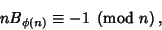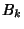## Giuga Number

Any Composite Numberwithfor all Prime Divisorsof.is a Giuga number Iffwhereis the Totient Function and Iffis a Giuga number Iffwhereis a Bernoulli Number andis the Totient Function. Every counterexample to Giuga's conjecture is a contradiction to Argoh's Conjecture and vice versa. The smallest known Giuga numbers are 30 (3 factors), 858, 1722 (4 factors), 66198 (5 factors), 2214408306, 24423128562 (6 factors), 432749205173838, 14737133470010574, 550843391309130318 (7 factors),

244197000982499715087866346, 554079914617070801288578559178(8 factors), ... (Sloane's A007850).

It is not known if there are an infinite number of Giuga numbers. All the above numbers have sum minus product equal to 1, and any Giuga number of higher order must have at least 59 factors. The smallest Odd Giuga number must have at least nine Prime factors.

Borwein, D.; Borwein, J. M.; Borwein, P. B.; and Girgensohn, R. Giuga's Conjecture on Primality.'' Amer. Math. Monthly 103, 40-50, 1996.
Sloane, N. J. A. Sequence A007850 in The On-Line Version of the Encyclopedia of Integer Sequences.'' http://www.research.att.com/~njas/sequences/eisonline.html.